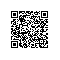# php语法

<strong>变量的定义：</strong>

$v1 = 1; echo$v1;

<strong>数据类型</strong>

“==”模糊等

“===”严格等

break

continue

function 函数名（形式参数1，形式参数2， 。。。。）

{

//函数体。。。。

return value;

}

function f1($s) { if($s>5)

{

return;

} else {

echo $s; return; }$v1 = $s *$s

}

function f2($a,$b, $c=2) {$s=$a+$b+$c; return$s;

substr的用法对比

js中：

var str1 = "abcdefg";

v1 = str1.substr(2,3); 从str1中的第2位置开始取3个。

php中：

$str1 = "abcdefg";$v1 = substr($str1, 2, 3); 从str1中的第2位置开始取3个。 其他方法详见手册 数学计算 详见手册 时间处理 获取时间（同C） 数组（array） php中，数组也是若干个数据的有序的排列成为一个整体。php的数组，其“下标”可以是数字，也可以是“字符串”，也可以是混合的。 如果全是数字下标，通常称为“索引数组” 如果是全是字符下标，则通常称为“关联数组” php中，通常不说“下标”这个概念，而是说“键”：键名和键值$arr1 = array ( 5,  “abc”,  ‘mm’=>11,  7=>70,  80,  “111”)

foreach (数组变量    as     $value) { 循环语句段 //此时$value就是每一个值

}

// 或者：

foreach (数组变量  as  $key =>$value)

{

循环语句段      //此时$key是每一个键， //$value是每一个值

}

php中：“全局”和“局部”是分离的，全局的只能在全局中用，局部的只能在局部中用。

global 变量名； //此句变量名为全局变量，且应该写在函数内部（局部）

php操作数据库

a) mysql_connect("数据库服务器名/ip", "登录名/用户名", "密码")

mysql_query("set names utf8;"); //mysql_query为一个php函数

mysql_query("use php0506_db;");

$result = mysql_query("增删改语句"); if（$result == true）

{

//执行成功之后的提示或其他语句

}

else

{

//执行成功之后的提示或其他语句

}

1， 连接数据库服务器

2， 设定编码

3， 选择要操作的数据库

4， 构建查询语句：$sql = “select * from liuyanbiao”; 5， 执行查询并返回结果：$rs = mysql_query($sql); 6， 判断$rs, 如果为真：

a)获取数据行数：$len = mysql_num_rows($rs);

b)for循环读取数据：$arr = mysql_fetch_array($rs );

i. 显示数据： echo $arr[‘字段名’]; 7， 判断$rs，如果为假：表示selec语句执行失败。

php项目介绍流程介绍：

php的其他知识：

get方式一：通过表单：

<form action=”文件名” method=”get”>

。。。。。。。。。。。。。。

</form>

get方式获取数据： $_GET[‘表单项的name值’] get方式二：通过链接： <a href=”文件名?name1=值1&name2=值2……” >文字</a> 这里，name1，name2等等只是一个数据项的名字，跟表单项类似（表单项的名字使用name属性） 例： <a href=”abc.php?n=123” >go1</a> <a href=”abc.php?kk=123&nn=abc” >go2</a> <a href=”abc.php?myname=123&pp=abc&x1=xyz” >go3</a> <a href="http://tuan.360.cn/?fname=hao_cai1_tuan_tuangou&amp;eee=hao_cai1_tuan_tuango">团购促销</a> get方式获取数据：$_GET[‘数据项名’]

php标签

Php是一门嵌入式，意味着代码的混编文件，需要将php代码从其他代码中区别出来，就有了php的标签。

<?php ?>

<script language=”php”>

</script>

<? ?>短标记

<% %>asp风格

Php的内置函数 unset()可以删除一个变量。

$hello = 'world'; echo$$name; world php的八种数据类型 整型 浮点 布尔 字符串 数组 对象 资源 NULL 定界符：Heredoc <<<标签 字符串内容 标签；$s1 = <<<STR

hello

STR;

<<<'标签'

$s4 = <<<'STR' world STR; 两种定界符的区别： Nowdoc不能解析内部的变量，而heredoc可以解析变量： 浮点数（双精度）的理论最大值是： 1.8E+308 类型的变量中常用的函数 var_dump();输出变量的信息（类型，值，长度） unset();删除一个变量，删除一个数组元素，甚至删除对象的属性。 lsset();判断一个变量是否存在，一个数组元素，一个对象属性是否存在。一个值是否为null。 empty();判断一个变量是否是空值。（0，‘’，array(),0.0, null都算什么都没有） 理解成 (bool) data 的反义词empty(data) Isset和empty的好处，在处理一个没有定义的变量时，不会报告一个notice级别的错误： PHP中的替代语法 替代流程控制中的{}，使用:替代{} if(10>20): echo 'yes'; else: echo 'no'; endif; 替代语句，不单单适用于if，switch，for，while。Foreach，都有相应的替代语法。 替代语法，常见于html和php的混编文件内。 如果只有一条语句，可以省略两端的大括号！如： if(10>20) echo 'yes'; else echo 'no'; 分开写的：else if else { if } 相当于，else语句中由于只有if语句而省略了语句快的大括号的。逻辑上与elseif一致。$score = 87;

if($score >= 90): echo 'A'; elseif($score >= 60):

echo 'B';

else:

echo 'C';

endif;

$score = 87; if($score >= 90):

echo 'A';

else:

if(\$score >= 60):

echo 'B';

else:

echo 'C';

endif;

endif;

include，require，include_once,require_once。使用钉钉扫一扫加入圈子
+ 订阅